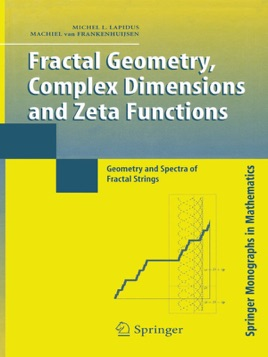• CHF 75.00

## Beschreibung des Verlags

Number theory, spectral geometry, and fractal geometry are interlinked in this in-depth study of the vibrations of fractal strings, that is, one-dimensional drums with fractal boundary.

Key Features

The Riemann hypothesis is given a natural geometric reformulation in the context of vibrating fractal strings

Complex dimensions of a fractal string, defined as the poles of an associated zeta function, are studied in detail, then used to understand the oscillations intrinsic to the corresponding fractal geometries and frequency spectra

Explicit formulas are extended to apply to the geometric, spectral, and dynamical zeta functions associated with a fractal

Examples of such explicit formulas include a Prime Orbit Theorem with error term for self-similar flows, and a geometric tube formula

The method of Diophantine approximation is used to study self-similar strings and flows

Analytical and geometric methods are used to obtain new results about the vertical distribution of zeros of number-theoretic and other zeta functions

Throughout new results are examined. The final chapter gives a new definition of fractality as the presence of nonreal complex dimensions with positive real parts, and discusses several open problems and extensions.

The significant studies and problems illuminated in this work may be used in a classroom setting at the graduate level. Fractal Geometry, Complex Dimensions and Zeta Functions will appeal to students and researchers in number theory, fractal geometry, dynamical systems, spectral geometry, and mathematical physics.

From Reviews of Fractal Geometry and Number Theory: Complex Dimensions of Fractal Strings and Zeros of Zeta Functions, by Michel Lapidus and Machiel van Frankenhuysen, Birkhäuser Boston Inc., 2000.

"This highly original self-contained book will appeal to geometers, fractalists, mathematical physicists and number theorists, as well as to graduate students in these fields and others interested in gaining insight into these rich areas either for its own sake or with a view to applications. They will find it a stimulating guide, well written in a clear and pleasant style."

–Mathematical Reviews

"It is the reviewer’s opinion that the authors have succeeded in showing that the complex dimensions provide a very natural and unifying mathematical framework for investigating the oscillations in the geometry and the spectrum of a fractal string. The book is well written. The exposition is self-contained, intelligent and well paced."

–Bulletin of the London Mathematical Society